# Smart Safe Box - SARS-CoV-2 Version

This project respects the norms of social distancing. Thus, the owner can communicate with the safe from any distance Great for this period!

IntermediateFull instructions provided18 hours34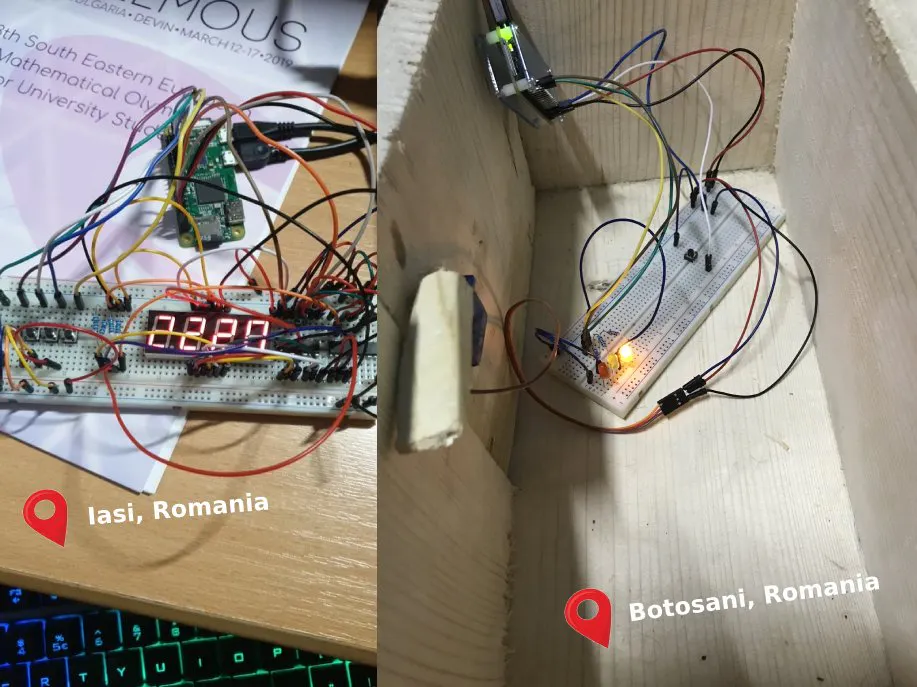## Things used in this project

### Hardware components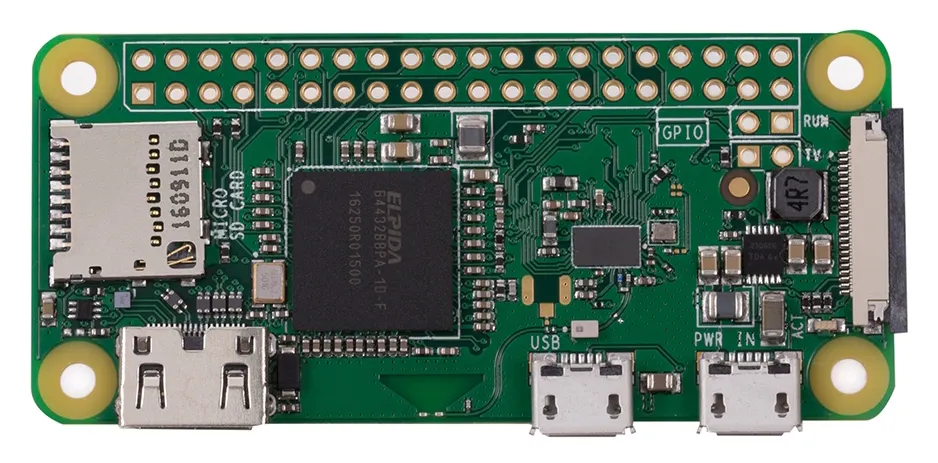Raspberry Pi Zero Wireless
×2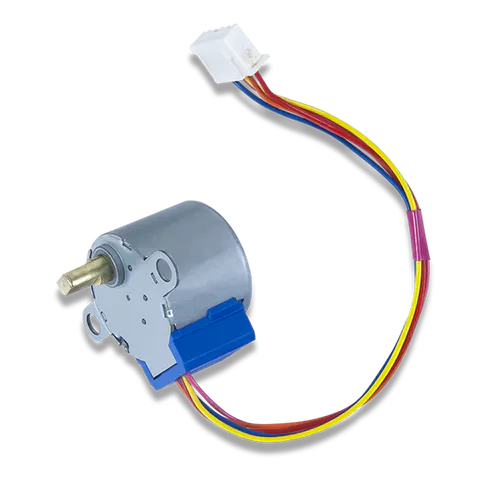Digilent Stepper Motor
×1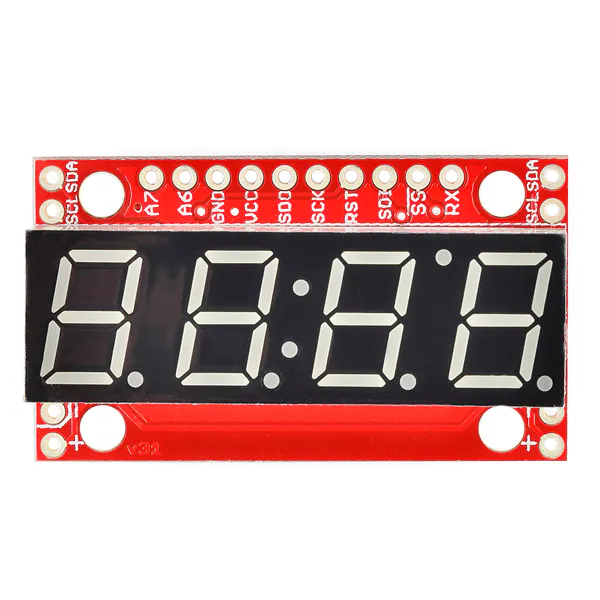SparkFun 7-Segment Serial Display - Red
×1SparkFun Pushbutton switch 12mm
×65 mm LED: Red
×1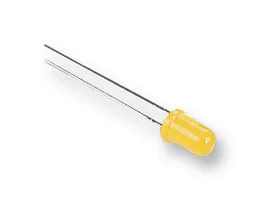5 mm LED: Yellow
×13 mm LED: Green
×1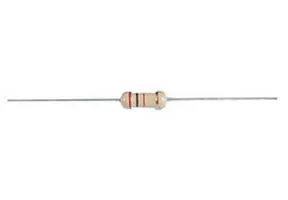Through Hole Resistor, 150 ohm
×7×2
 74HC595 Shift Register (DIP-16)
×2
 Male/Male Jumper Wires
×42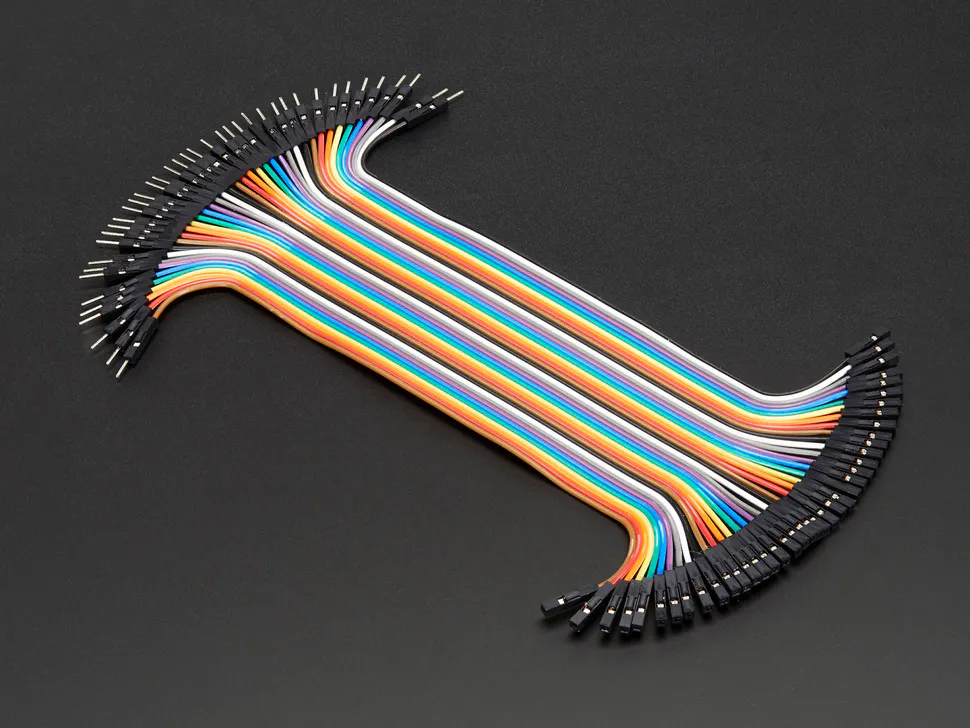Male/Female Jumper Wires
×20

### Software apps and online servicesRaspberry Pi Raspbian
 Kafka - Message Broker
 Cloud VPS

## Schematics

### Led Schematic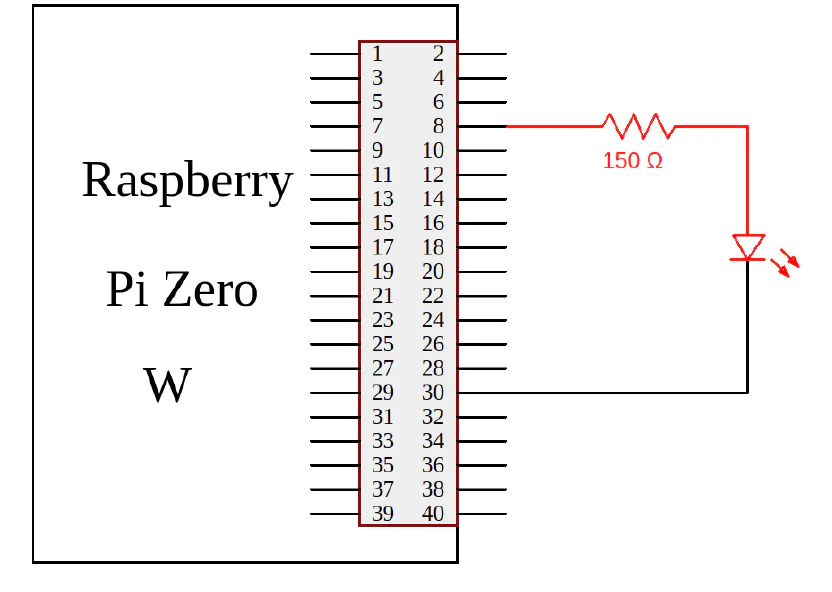### Led Visual Representation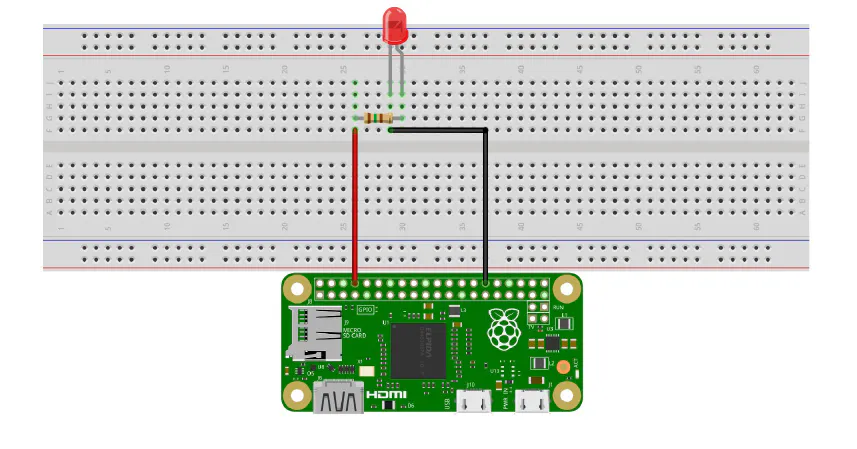### Servomotor Schematic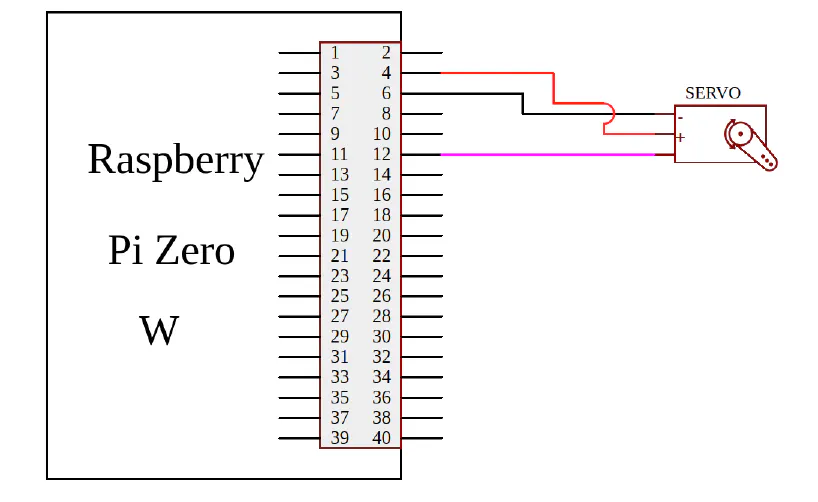### Button Schematic

This is the basic schematic for buttons. In our project we used 4 buttons conencted like this.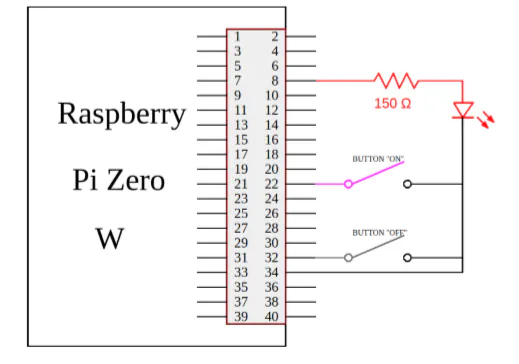### Button Visual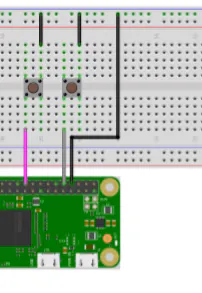### Display Schematic

This is the scheme that was provided with the display and which we used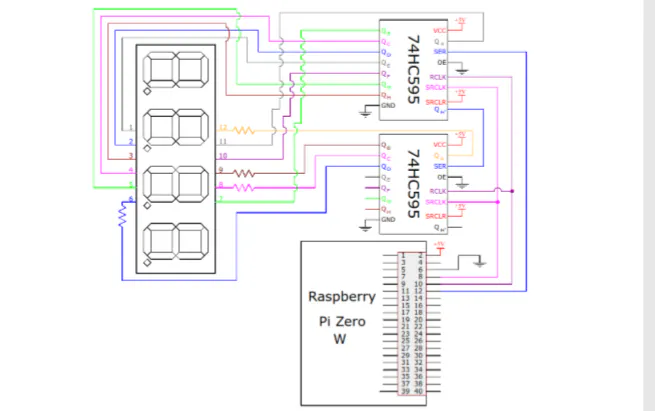### Display Visual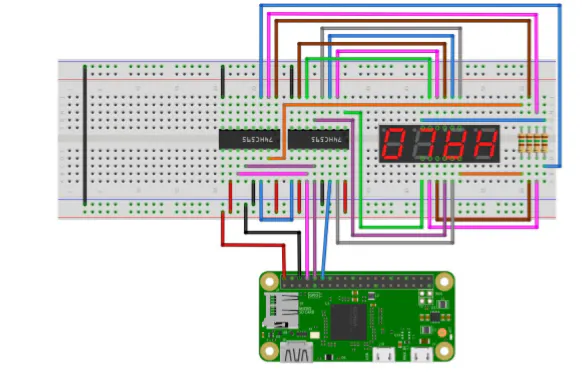### Servomotor Visual Representation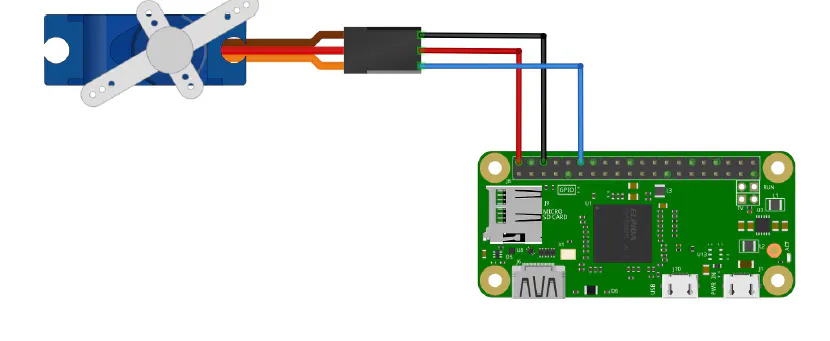## Code

### safe.py

Python
This code represents the logic for safe. It is also a client for kafka server that runs on Cloud VPS. In this code you can see the consumer that read the password, the function for stepper movement, and the logic for leds.
```#import the libraries
import pigpio
import time
import RPi.GPIO as GPIO
import ctypes
from kafka import KafkaConsumer

#creating consumer for getting messages from password topic
result_consumer = KafkaConsumer(topic, bootstrap_servers='PUT_HERE_PUBLIC_SERVER_IP')

GPIO.setmode(GPIO.BOARD)

pi = pigpio.pi()

#store the pin used by the servo
servo = 18

#set the pin as OUTPUT
pi.set_mode(servo, pigpio.OUTPUT)

#position for closed safe
closed = 500

#position for open safe
opened = 1500

#define the pin used for button close safe
buttonClose = 7

#define the pin used for red led
redLed = 37

#define the pin used for green led
greenLed = 35

#define the pin used for yellow led
yellowLed = 33

#set the pin for the led as OUTPUT
GPIO.setup(redLed, GPIO.OUT)
GPIO.setup(greenLed, GPIO.OUT)
GPIO.setup(yellowLed, GPIO.OUT)

shouldClose = False

#set the pins for the buttons as INPUT, and we will
#set the initial value to On, or we can say that will be pulled up
GPIO.setup(buttonClose, GPIO.IN, pull_up_down = GPIO.PUD_UP)

#function for opening the safe
def open_safe():
#put the servo in the position open position
pi.set_servo_pulsewidth(servo, opened)
time.sleep(1)

#function for closing the safe
def close_safe():
#put the servo in the closed position
pi.set_servo_pulsewidth(servo, closed)
#sleep 1 second
time.sleep(1)

global should_close
while shouldClose == False:
message = next(result_consumer)

#function that raise exception to close the thread
if res > 1:
print('Exception raise failure')

#turn on the yellow Led
GPIO.output(yellowLed, GPIO.HIGH)
#turn off the green Led
GPIO.output(greenLed, GPIO.LOW)
#turn off the red Led
GPIO.output(redLed, GPIO.LOW)

try:
while True:
#turn on the green Led
GPIO.output(greenLed, GPIO.HIGH)
#turn off the red Led
GPIO.output(redLed, GPIO.LOW)
#turn off the yellow Led
GPIO.output(yellowLed, GPIO.LOW)
#check if safe it is already opened
if pi.get_servo_pulsewidth(servo) != opened:
open_safe()
else:
if pi.get_servo_pulsewidth(servo) == opened:
else:
#turn on the red Led
GPIO.output(redLed, GPIO.HIGH)
#turn off the green Led
GPIO.output(greenLed, GPIO.LOW)
#turn off the yellow Led
GPIO.output(yellowLed, GPIO.LOW)

#check close button pressed
if GPIO.input(buttonClose) == GPIO.LOW:
#turn on the yellow Led
GPIO.output(yellowLed, GPIO.HIGH)
#turn off the green Led
GPIO.output(redLed, GPIO.LOW)
#turn off the red Led
GPIO.output(greenLed, GPIO.LOW)
#check if safe it is already closed
if pi.get_servo_pulsewidth(servo) != closed:
except KeyboardInterrupt:
shouldClose = True
#wait for thread to finish work
#stop the servo pulses
pi.set_servo_pulsewidth(servo, 0)
#stop the connection with the daemon
pi.stop()
#clean all the used ports
GPIO.cleanup()
#close consumer
result_consumer.close()
```

### buttons.py

Python
This is the code for the board that has the 7 digits display and the input buttons for the password. We have the client for the kafka, the initialization for the buttons and the code for the display. An example code of the display was given with the materials, and we modified the code so it could show different digits on each position at the same time.
```#import the libraries used
import time
import RPi.GPIO as GPIO
import sys
from kafka import KafkaProducer

#kafka variables

#we will set the pin numbering to the GPIO.BOARD numbering
GPIO.setmode(GPIO.BOARD)

#define the pins used
#Buttons
button1 = 35
button2 = 33
button3 = 31
button4 = 29
buttonVALIDATE = 37

#LCD
dataPin = 12
latchPin = 10
clockPin = 8

cifra1 = 0
cifra2 = 0
cifra3 = 0
cifra4 = 0

p1 = False
p2 = False
p3 = False
p4 = False
pValidate = False

#set the pins for the buttons as INPUT, and we will
#set the initial value to On, or we can say that will be pulled up
#Buttons
GPIO.setup(button1, GPIO.IN, pull_up_down = GPIO.PUD_UP)
GPIO.setup(button2, GPIO.IN, pull_up_down = GPIO.PUD_UP)
GPIO.setup(button3, GPIO.IN, pull_up_down = GPIO.PUD_UP)
GPIO.setup(button4, GPIO.IN, pull_up_down = GPIO.PUD_UP)
GPIO.setup(buttonVALIDATE, GPIO.IN, pull_up_down = GPIO.PUD_UP)

#LCD
GPIO.setup(dataPin, GPIO.OUT)
GPIO.setup(latchPin, GPIO.OUT)
GPIO.setup(clockPin, GPIO.OUT)
#set the initial state for the pins as LOW
GPIO.output(dataPin, GPIO.LOW)
GPIO.output(latchPin, GPIO.LOW)
GPIO.output(clockPin, GPIO.LOW)

#create byte variables that have only one segment set to HIGH
a = 0b00000001
b = 0b00000010
c = 0b00000100
d = 0b00001000
e = 0b00010000
f = 0b00100000
g = 0b01000000
dot = 0b10000000

#declare variables that will be send to the first shift
#register and turn on the LED segments and show a digit
#from 0 to 9, with or without the dot
zero = 191         #0b10111111
zero_no_dot = 63   #0b00111111
one = 134          #0b10000110
one_no_dot = 6     #0b00000110
two = 219          #0b11011011
two_no_dot = 91    #0b01011011
three = 207        #0b11001111
three_no_dot = 79  #0b01001111
four = 230         #0b11100110
four_no_dot = 102  #0b01100110
five = 237         #0b11101101
five_no_dot = 109  #0b01101101
six = 253          #0b11111101
six_no_dot = 125   #0b01111101
seven = 135        #0b10000111
seven_no_dot = 7   #0b00000111
eight = 255        #0b11111111
eight_no_dot = 127 #0b01111111
nine = 239         #0b11101111
nine_no_dot = 111  #0b01101111

numbers=[zero_no_dot,one_no_dot,two_no_dot,three_no_dot,
four_no_dot,five_no_dot,six_no_dot,
seven_no_dot,eight_no_dot,nine_no_dot,
dot]

#declare a variable that will store the current digits active
digit = 0

def Digit(x):
global digit
if x == 1:
digit = 14 #0b00001110
elif x == 2:
digit = 13 #0b00001101
elif x == 3:
digit = 11 #0b00001011
elif x == 4:
digit = 7 #0b00000111
elif x == 5:
digit = 0 #0b00000000

#function to send the values to the shift registers
def shift(buffer):
#make the global variable available
global digit

#send the bits to the second shift register
for i in range(0,8):
GPIO.output(dataPin, (128 & (digit << i)))
GPIO.output(clockPin, GPIO.HIGH)
time.sleep(0.00005)
GPIO.output(clockPin, GPIO.LOW)

#send the bits to the first shift register
for i in range(0,8):
GPIO.output(dataPin, (128 & (buffer << i)))
GPIO.output(clockPin, GPIO.HIGH)
time.sleep(0.00005)
GPIO.output(clockPin, GPIO.LOW)

#shift the bits
GPIO.output(latchPin, GPIO.HIGH)
time.sleep(0.00005)
GPIO.output(latchPin, GPIO.LOW)

def refresh():
global cifra1
global cifra2
global cifra3
global cifra4
global numbers
Digit(1)
shift(numbers[cifra1])
Digit(2)
shift(numbers[cifra2])
Digit(3)
shift(numbers[cifra3])
Digit(4)
shift(numbers[cifra4])

def checkButtons():
global cifra1
global cifra2
global cifra3
global cifra4
global p1
global p2
global p3
global p4
global pValidate

while shouldClose == False:
#check if the 1 button was pressed
if GPIO.input(button1) == GPIO.LOW:
#turn on the Led
if p1 == False:
cifra1 = (cifra1+1)%10
p1 = True
else:
p1 = False

#check if the 2 button was pressed
if GPIO.input(button2) == GPIO.LOW:
#turn off the Led
if p2 == False:
cifra2 = (cifra2+1)%10
p2 = True
else:
p2 = False

#check if the 3 button was pressed
if GPIO.input(button3) == GPIO.LOW:
#turn off the Led
if p3 == False:
cifra3 = (cifra3+1)%10
p3 = True
else:
p3 = False

#check if the 4 button was pressed
if GPIO.input(button4) == GPIO.LOW:
#turn off the Led
if p4 == False:
cifra4 = (cifra4+1)%10
p4 = True
else:
p4 = False

#check if the VALIDATE button was pressed
if GPIO.input(buttonVALIDATE) == GPIO.LOW:
#turn off the Led
if pValidate == False:
pValidate = True
password = cifra1.__str__() + cifra2.__str__() + cifra3.__str__() + cifra4.__str__()

#send password via kafka to the door

#reset digits
cifra1 = 0
cifra2 = 0
cifra3 = 0
cifra4 = 0
else:
pValidate = False

time.sleep(0.1)

shouldClose = False

try:
#call the "Digit" function in order to update
#the value of the "digit" variable
Digit(5)

#send two byte values tothe shift registers
#after this, all the A LEDs will turn on, because
#all the digits are turned on (they are set to LOW)
shift(dot)
time.sleep(1)

while True:
refresh()

except KeyboardInterrupt:
pass
shouldClose = True

digit = 0
shift(0)
#clean all the used ports
GPIO.cleanup()
```

## Credits

### Alexandru Baciu

1 project • 0 followers

### Marian Haralamb

1 project • 0 followers